Courses

# Study Notes for Number Systems Electrical Engineering (EE) Notes | EduRev

## Electrical Engineering (EE) : Study Notes for Number Systems Electrical Engineering (EE) Notes | EduRev

The document Study Notes for Number Systems Electrical Engineering (EE) Notes | EduRev is a part of Electrical Engineering (EE) category.
All you need of Electrical Engineering (EE) at this link: Electrical Engineering (EE)

Binary Codes

• Binary codes are codes which are represented in a binary system with modification from original ones.
• In general, N bits can represent up to 2N distinct values.
• Conversely, to represent a range of M values, the number of bits required is [log2 M].

1 bit → represents up to 2 values (0, 1)
2 bits → represents up to 4 values (00, 01, 10, 11)
3 bits  → represents up to 8 values (000, 001, 010, 011, 100, 101, 110, 111)
4 bits  → represents up to 16 values (0000, 0001, 0010, ..., 1110, 1111)
32 values  → requires 5 bits
40 values  → requires 6 bits
64 values  → requires 6 bits
100 values  →  requires 7 bits
1024 values → requires 10 bits

• The base or radix of a number system is the number of digits present. The decimal numeral system has a base or radix of 10, where the set of 10 symbols (digits) is {0, 1, 2, 3, 4, 5, 6, 7, 8, 9}. The weights are in powers of ten.
• In general, a bases-b number (an an-1 … a0 . f1 f2 … fm)b has the value
(an × bn) + (an-1 × bn-1) +…+ (a0 × b0) + (f1 × b-1) + (f2 × b-2) +…+ (fm × b-m)
• Weighted Binary System: Weighted binary codes are those which obey the positional weighting principles, each position of a number represents a specific weight.
e.g., 8421, 2421, 5211
• Sequential Code: A code is said to be sequential when two subsequent codes, seen as numbers in the binary representation, differ by one. The 8421 and excess-3 codes are sequential, whereas 2421 and 5211 codes are not.
• Non-weighted Codes: Non-weighted codes are codes that are not positionally weighted. That is each position within the binary number is not assigned a fixed value.
• Reflective Code: A code is said to be reflective when the code for 9 is a complement for the code for 0 and so is for 8 and 1 codes, 7 and 2, 6 and 3, 5 and 4. Codes 2421, 5211 and excess-3 are reflective, whereas the 8421 code is not.

BCD (Binary Coded Decimal)

• It is a straight assignment of the binary equivalent. To encode a decimal number using the common BCD encoding. Each decimal digit is stored in a 4-bit number.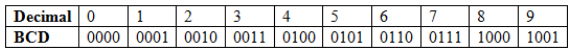• BCD encoding for number 127 would be
1 2 7
(0001 0010 0111) → BCD equivalent of 127
whereas the pure binary number would be (01111111)2
• BCD
Add (148 + 157) = ?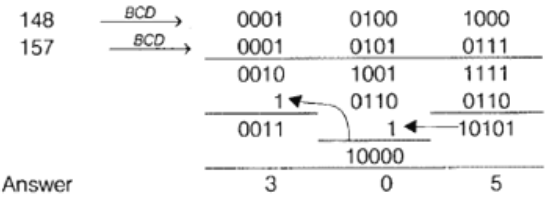When the sum of 2 digits is greater than or equal to 9, then we need to add 6 i.e., 0110.

• 2421 Code
This is a weighted code, its weights are 2, 4, 2 and 1. A decimal number is represented in 4-bit form and the total 4 bits weight is
2 + 4 + 2 + 1 = 9.
Hence, 2421 code represents the decimal numbers from 0 to 9.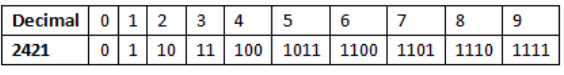• Excess-3 Code
Excess-3 is a non-weighted code used to represent decimal numbers. The code derives its name from the fact that each binary code is the corresponding 8421 code plus 0011 (3).
e.g.,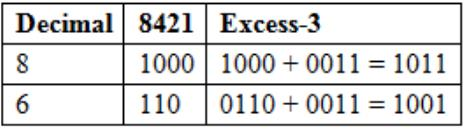• Gray Code
This is a variable weighted code and is cyclic. This means that it is arranged so that every transition from one value to the next value involves only one-bit Change.
• Binary to Gray Code Conversion
1. Write down the number in binary codes.
2. The Most Significant Bit (MSB) of the gray code will be same as the MSB of binary code.
3. Perform XOR operation on MSB and next bit to the MSB in a binary number.
4. Repeat step 3 till all bits of the binary number have been XO Red, the resultant code is the gray code equivalent to the binary code.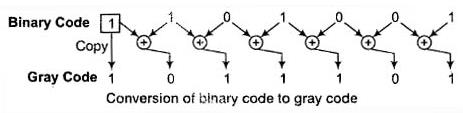• Gray Code to Binary Conversion
2. Copy this bit as the MSB of the binary number.
3. Now, perform Ex-OR operation of this bit with the next bit of the binary number.
4. Repeat step 3 till all bits of gray coded number have been used in XOR operation. The resultant number is the binary equivalent of the gray number.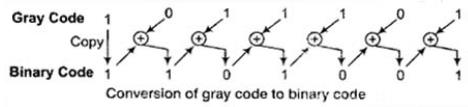Complements

• Complements are used in a digital computer system for simplifying the subtraction operation and for logical manipulation.
• There are two types of complements for each base r system,
1. The r′ s complement
2. The (r′ – 1)′s complement
• The r's Complement
Given a positive number N with base r with an integer part of n digits. The r's complement of N is defined as rn – N for N ≠ 0 and 0 for N = 0.
e.g., 10's complement of (25.639)10 is (102 - 25.639)
100 - 25.639 = 74 – 361, here the number of digits in integer part is 2
means n = 2
• The (r – 1)′s Complement
Given, a positive number N in base r with an integer part of n digits and a fraction part of m digits, the (r – 1)'s complement of N is defined as rn – r–m – N.
• 1’s complement of (52520)10 is (105 – 1 – 52520)
= 99999 – 52520 = 47479
Because number of integer part is 5, so rn = 105 and no fractional part is
Present' so r–m = 10–0 = 1
• 1’s complement of (0.3267)10 is (100 – 10–4 – 0.3267)
= 1 – 0.0001 – 0.3267
= 0.9999 – 0.3267 = 0.6732
No integer part, so 10n = 100 = 1
• 1’s complement of (101100)2 is (26 – 20)10 – (101100)2
= (64 – 1)10 – (101100)2
= (63)10 = (101100)2
= 111111 – 101100 = 010011
• Key Points
• 520 → Here, n = 3, but (052) → here n = 2.
• In the latter example, 0 is of no significance.

Representation of Integers

• The are three possible ways to represent a number
1. Signed magnitude method
2. 1's complement method
3. 2's complement method
• Signed Magnitude Method
• The number is divided into two parts, one is sign bit and another part for magnitude, In the example we are using 5-bit register to represent –6 and +6.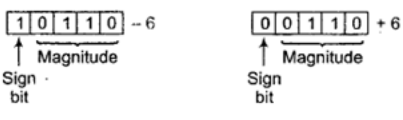• The range of Number For n bit register, MSB will be a sign bit and (n – 1) bits will be magnitude.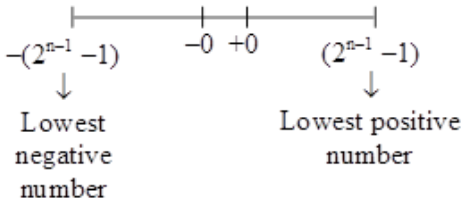Key Points

• The drawback of signed magnitude method is that 0 will be having 2 different representation one will be 10000 i.e., –0 and the other one will be 00000 + 0.

1's Complement Method

• Positive numbers are represented in the same way as in sign magnitude method. If the number is negative, then it is represented using 1's complement method. For this, we first need to represent the number with a positive sign and then take 1's complement of this number.
• e.g., Suppose we are using a 5-bit register. The representation of –6 will be as below.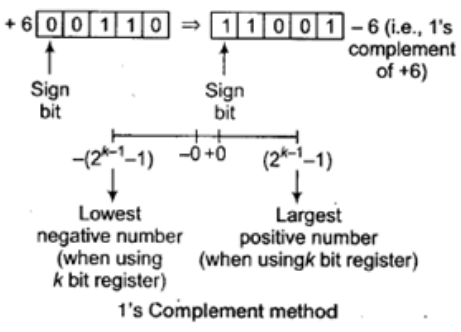• Key Points
• The only drawback of 1's complement method is that there are two different representation for zero, one is –0 and other is +0.

2's Complement Method

• Positive numbers are represented in the same way as in sign magnitude. For representing a negative number, we take 2's complement of the corresponding positive number.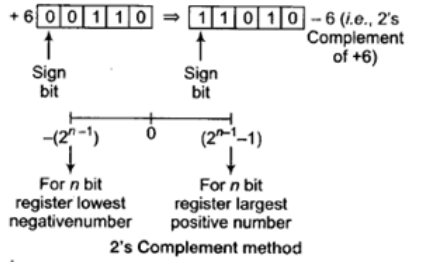Properties of 2's Complement

• 2's complement representation allows the use of binary arithmetic operations on signed integers, yielding the current 2's complement result.
• Positive Numbers Positive 2's complement numbers are represented as the simple binary.
• Negative Numbers Negative 2's complement numbers are represented as the binary number that when added to a positive number of the same magnitude equals zero.
Offer running on EduRev: Apply code STAYHOME200 to get INR 200 off on our premium plan EduRev Infinity!

,

,

,

,

,

,

,

,

,

,

,

,

,

,

,

,

,

,

,

,

,

;